[back to Cosine Kitty's math page]

I made an interesting discovery today (29 January 2005). I realized that it is possible to look at the Mandelbrot Set in a different way. Usually it is graphed by iterating the formula

z' = z2 + c

and coloring each complex-valued pixel c based on how long it takes to escape a circle of radius 2. The resulting image looks like this: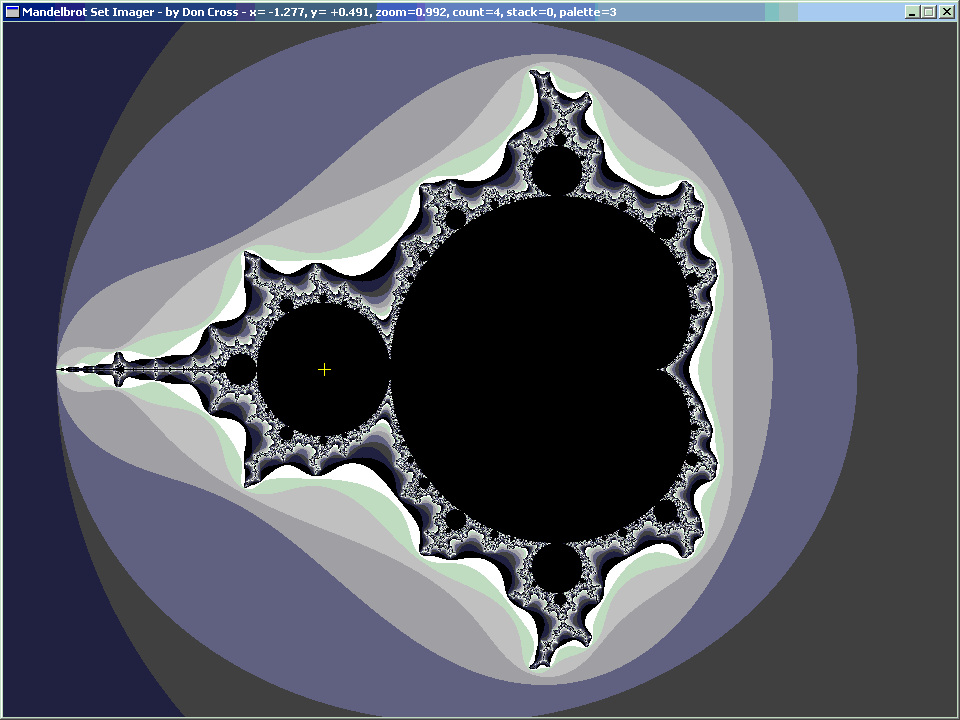Recently I have been studying the orbital period of various points inside the Mandelbrot Set, meaning the number of steps it takes to cycle back to the same spot once you have iterated a given c value enough times for it to "settle down" into a cycle. For example, if you choose c = −1 (marked with yellow cross-hairs in the image above), the orbital period is 2, because:

 z = 0 z = z2 + c = 02 + (−1) = −1 z = z2 + c = (−1)2 + (−1) = 0 z = z2 + c = 02 + (−1) = −1 z = z2 + c = (−1)2 + (−1) = 0 ...

My own home-brew Mandelbrot Set imager now allows me to type in an arbitrary algebraic expression f(z) in terms of the complex variable z, and generates a fractal based on iterating Newton's Method to find a solution for f(z)=0.

It occurred to me that it would be interesting to graph solutions for various orbital periods in the Mandelbrot formula. For example, if I wanted to find places where the Mandelbrot formula repeated every iteration (i.e. orbital period = 1), I would solve for z2 + z = z, or z2 = 0. Of course, this is a trivial case, since it is obvious that the only solution is z=0.

As one might expect, a trivial formula yields a trivial image, in this case not even a fractal... just a set of concentric circles:z2 + z = z
[Orbital Period = 1]
 Re(z) Im(z) 0 0

For the orbital period of 2, we get our first non-trivial image. This time it is a fractal. The solutions are z = +1 and z = −1, which show up as the larger horizontal eyes. This is interesting, but there is no resemblance of the Mandelbrot Set (yet).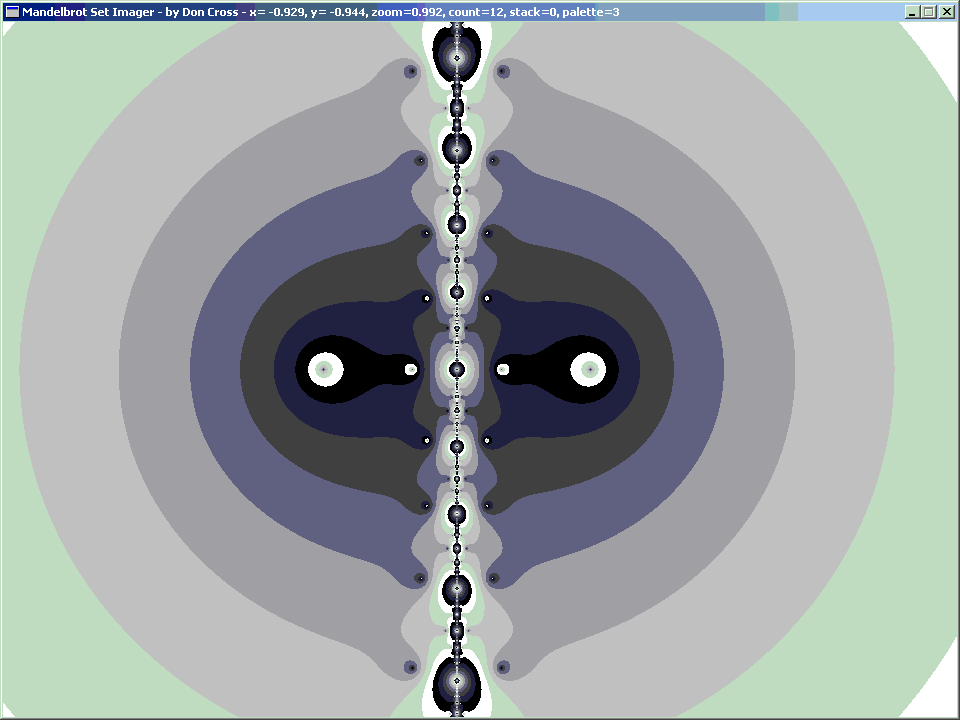(z2 + z)2 + z = z
[Orbital Period = 2]
 Re(z) Im(z) 0 0 −1 0

Now at orbital period 3, something interesting happens: we are starting to see a definite pear shape.

Also, because of the Fundamental Theorem of Algebra, there are 2p−1 complex solutions, where p is the orbital period, because each higher orbital period corresponds with a polynomial based on squaring the previous period's polynomial. The reason it is 2p−1 and not 2p is because when the orbital period is 1, there is only 1 solution, and 1 = 20.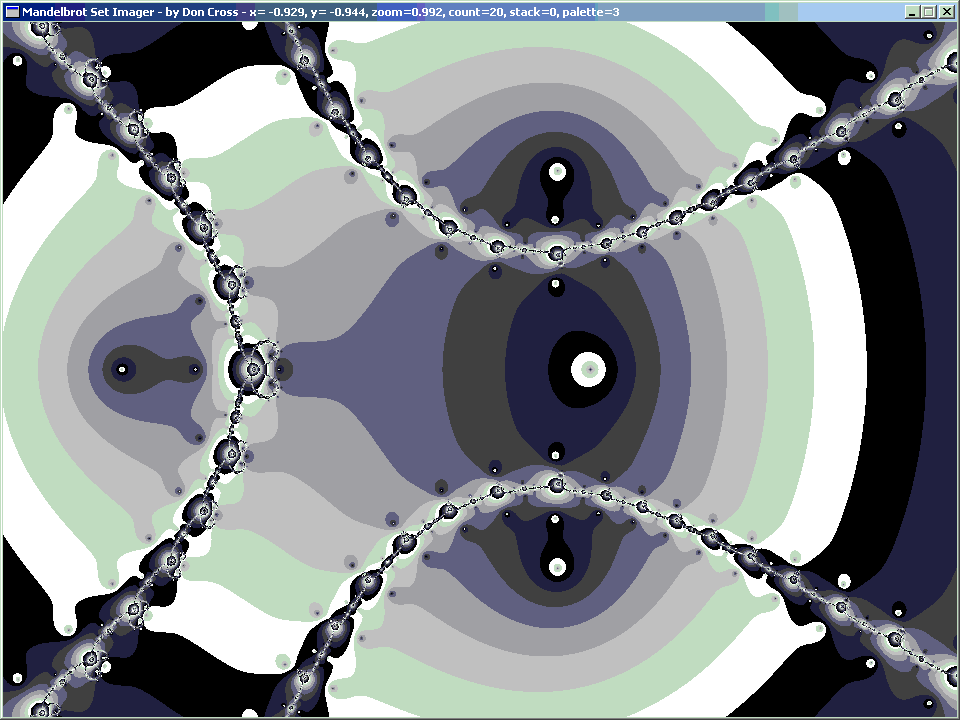((z2 + z)2 + z)2 + z = z
[Orbital Period = 3]
 Re(z) Im(z) 0 0 −1.75487767 0 −0.12256117 ±0.74486177(((z2 + z)2 + z)2 + z)2 + z = z
[Orbital Period = 4]
 Re(z) Im(z) 0 0 −1 0 −1.31070264 0 −1.94079981 0 0.28227139 ±0.53006062 −0.15652017 ±1.03224711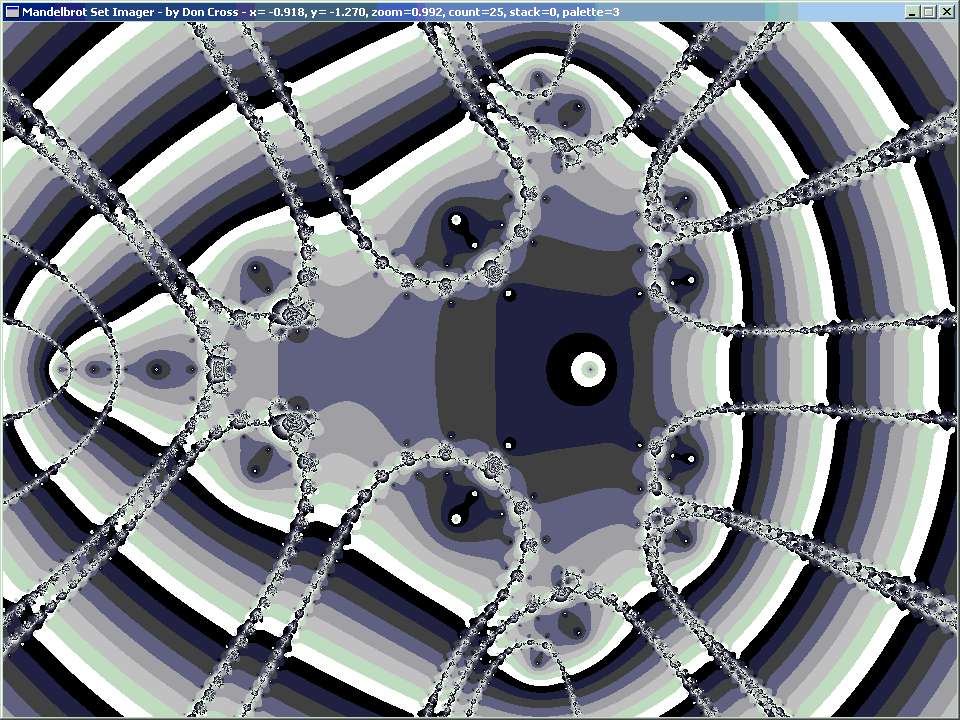((((z2 + z)2 + z)2 + z)2 + z)2 + z = z
[Orbital Period = 5]
 Re(z) Im(z) 0 0 −1.62541373 0 −1.86078252 0 −1.98542425 0 0.37951359 ±0.33493231 0.35925922 ±0.64251374 −0.04421236 ±0.98658098 −0.19804210 ±1.10026954 −0.50434018 ±0.56276576 −1.25636793 ±0.38032096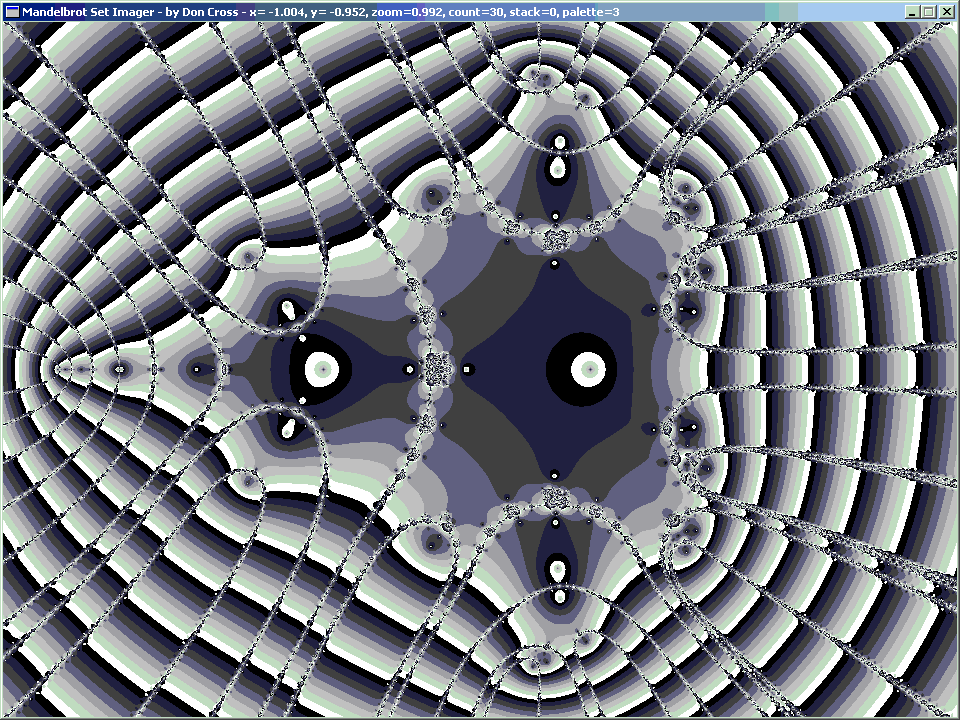(((((z2 + z)2 + z)2 + z)2 + z)2 + z)2 + z = z
[Orbital Period = 6]
 Re(z) Im(z) 0 0 −1 0 −1.47601464 0 −1.75487767 0 −1.77289290 0 −1.90728009 0 −1.96677322 0 −1.99637614 0 0.38900684 ±0.21585065 0.44332563 ±0.37296242 0.39653457 ±0.60418181 0.35989274 ±0.68476202 −0.01557039 ±1.02049737 −0.11341866 ±0.86056947 −0.12256117 ±0.74486177 −0.16359826 ±1.09778064 −0.21752675 ±1.11445427 −0.59689164 ±0.66298074 −1.13800067 ±0.24033240 −1.28408496 ±0.42726890((((((z2 + z)2 + z)2 + z)2 + z)2 + z)2 + z)2 + z = z [Orbital Period = 7]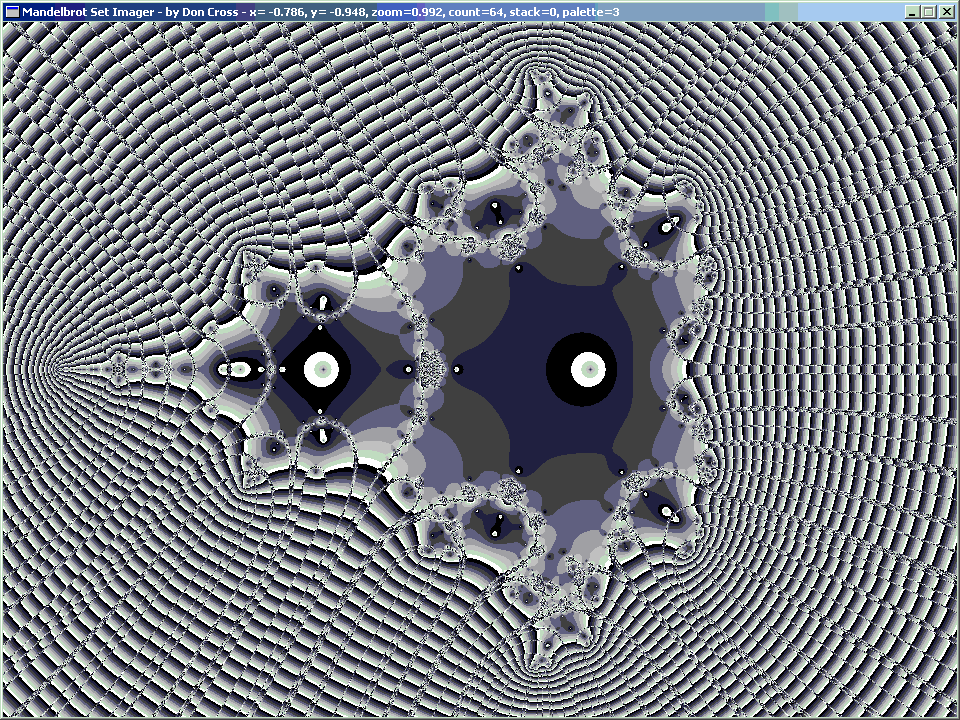(((((((z2 + z)2 + z)2 + z)2 + z)2 + z)2 + z)2 + z)2 + z = z [Orbital Period = 8]